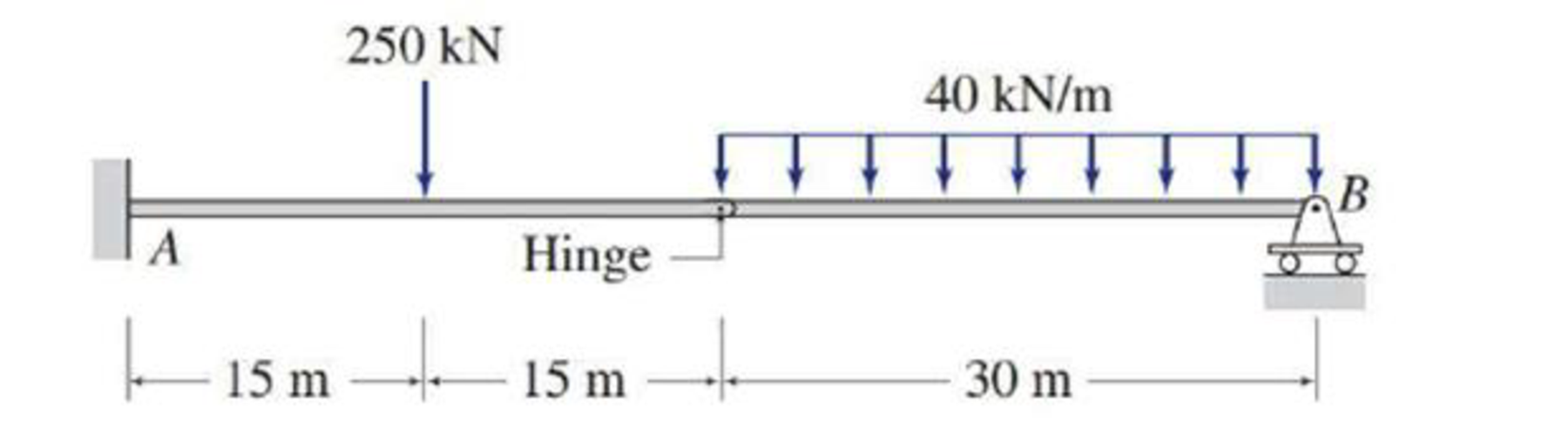# Determine the reactions at the supports for the structures shown. FIG.P3.28

#### Solutions

Chapter
Section
Chapter 3, Problem 28P
Textbook Problem
5 views

## Determine the reactions at the supports for the structures shown.FIG.P3.28

To determine

Calculate the support reactions for the given structure.

### Explanation of Solution

Given information:

The structure is given in the Figure.

Apply the sign conventions for calculating reaction forces and moments using the three equations of equilibrium as shown below.

• For summation of forces along x-direction is equal to zero (Fx=0), consider the forces acting towards right side as positive (+) and the forces acting towards left side as negative ().
• For summation of forces along y-direction is equal to zero (Fy=0), consider the upward force as positive (+) and the downward force as negative ().
• For summation of moment about a point is equal to zero (Matapoint=0), consider the clockwise moment as negative and the counter clockwise moment as positive.

Calculation:

Let Ax, Ay, and MA be the horizontal reaction, vertical reaction and moment at the fixed support A.

Let By be the vertical reaction at the roller support B.

Sketch the free body diagram of the structure as shown in Figure 1.

Use equilibrium equations:

For the member BC, the summation of moments about C is equal to 0.

MCBC=040(30)(302)+By(30)=030By=18,000By=600kN

Therefore, the vertical reaction at B is By=600kN_.

Summation of moments about A is equal to 0.

MA=0MA250(15)(40)(30)(45)+By(60)=0

Substitute 600 kN for By

### Still sussing out bartleby?

Check out a sample textbook solution.

See a sample solution

#### The Solution to Your Study Problems

Bartleby provides explanations to thousands of textbook problems written by our experts, many with advanced degrees!

Get Started

Find more solutions based on key concepts
Determine the load that can be lifted by the hydraulic system shown in the accompanying figure. All of the nece...

Engineering Fundamentals: An Introduction to Engineering (MindTap Course List)

Is batch processing still relevant? Why or why not?

Systems Analysis and Design (Shelly Cashman Series) (MindTap Course List)

Determine the clamping force at A due to the 15-lb horizontal force applied to the handle at E.

International Edition---engineering Mechanics: Statics, 4th Edition

If you were to interview a candidate for a technology-related position, what types of questions would you ask?

Enhanced Discovering Computers 2017 (Shelly Cashman Series) (MindTap Course List)

What types of tip seals are used with cutting torch tips?

Welding: Principles and Applications (MindTap Course List)

If your motherboard supports ECC DDR3 memory, can you substitute non-ECC DDR3 memory?

A+ Guide to Hardware (Standalone Book) (MindTap Course List)